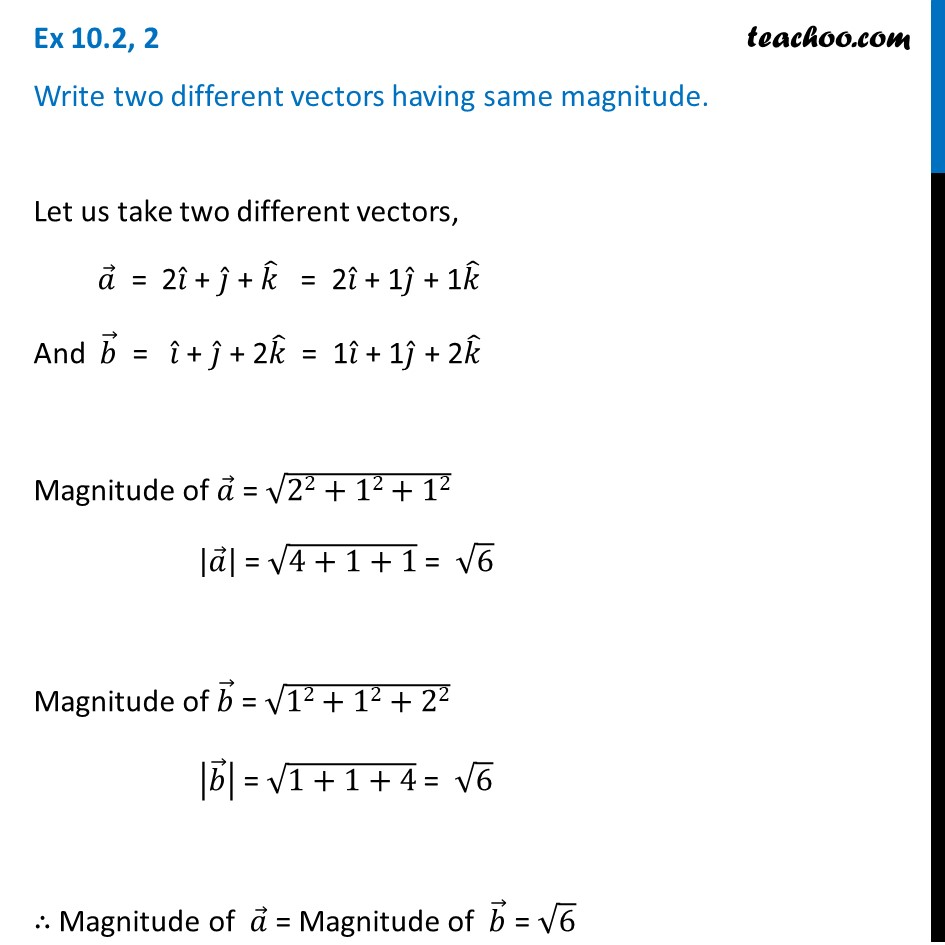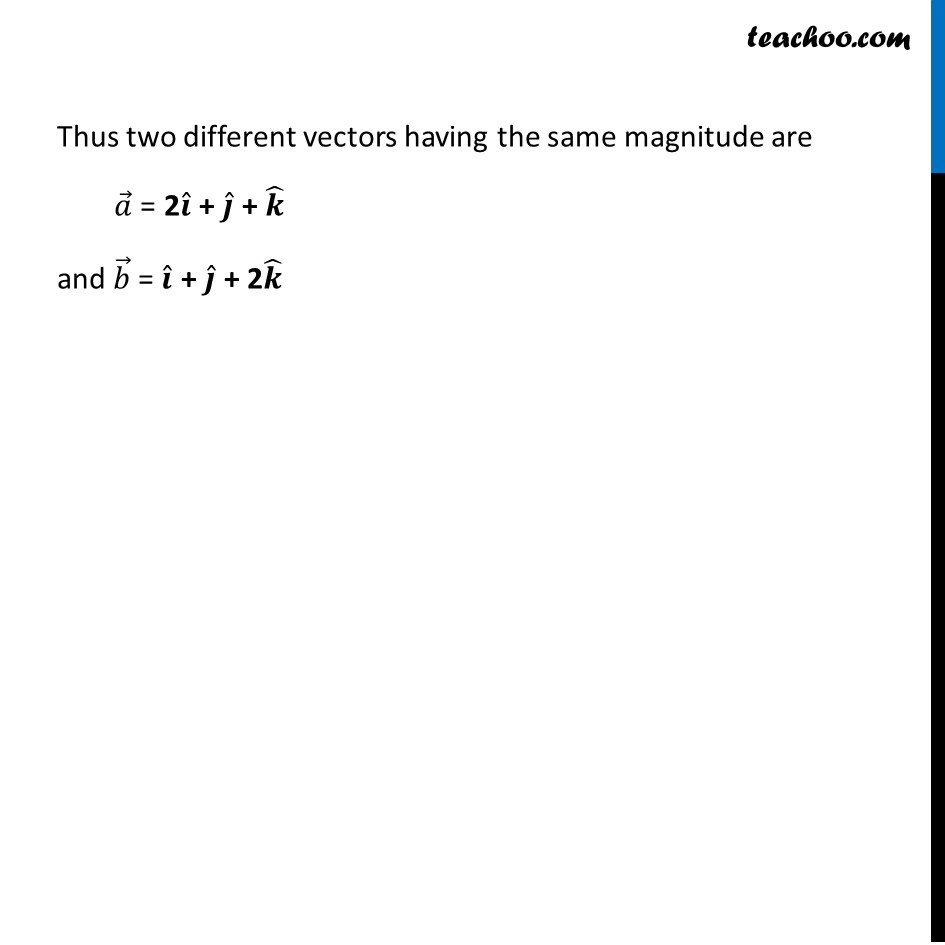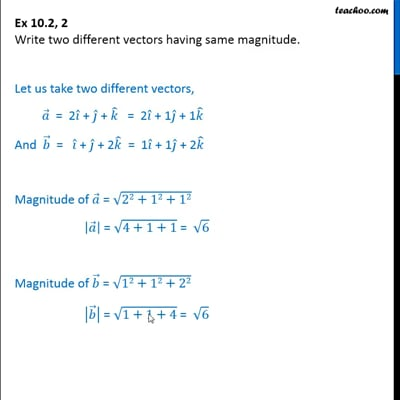Ex 10.2

Chapter 10 Class 12 Vector Algebra
Serial order wiseThis video is only available for Teachoo black users

Introducing your new favourite teacher - Teachoo Black, at only ₹83 per month

### Transcript

Ex 10.2, 2 Write two different vectors having same magnitude. Let us take two different vectors, 𝑎 ⃗ = 2𝑖 ̂ + 𝑗 ̂ + 𝑘 ̂ = 2𝑖 ̂ + 1𝑗 ̂ + 1𝑘 ̂ And 𝑏 ⃗ = 𝑖 ̂ + 𝑗 ̂ + 2𝑘 ̂ = 1𝑖 ̂ + 1𝑗 ̂ + 2𝑘 ̂ Magnitude of 𝑎 ⃗ = √(22+12+12) |𝑎 ⃗ | = √(4+1+1) = √6 Magnitude of 𝑏 ⃗ = √(12+12+22) |𝑏 ⃗ | = √(1+1+4) = √6 ∴ Magnitude of 𝑎 ⃗ = Magnitude of 𝑏 ⃗ = √6 Thus two different vectors having the same magnitude are 𝑎 ⃗ = 2𝒊 ̂ + 𝒋 ̂ + 𝒌 ̂ and 𝑏 ⃗ = 𝒊 ̂ + 𝒋 ̂ + 2𝒌 ̂